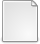# A New Scaling and Squaring Algorithm for the Matrix Exponential

Al-Mohy, Awad H. and Higham, Nicholas J. (2009) A New Scaling and Squaring Algorithm for the Matrix Exponential. SIAM Journal On Matrix Analysis and Applications., 31 (3). pp. 970-989. ISSN 1095-7162PDF alhi09a.pdf Download (281kB)Other expm_new.zip Download (5kB)
Official URL: http://scitation.aip.org/simax

## Abstract

The scaling and squaring method for the matrix exponential is based on the approximation $e^A \approx (r_m(2^{-s}A))^{2^s}$, where $r_m(x)$ is the $[m/m]$ Pad\'e approximant to $e^x$ and the integers $m$ and $s$ are to be chosen. Several authors have identified a weakness of existing scaling and squaring algorithms termed overscaling, in which a value of $s$ much larger than necessary is chosen, causing a loss of accuracy in floating point arithmetic. Building on the scaling and squaring algorithm of Higham [{\em SIAM J. Matrix Anal. Appl.}, 26\penalty0 (4):\penalty0 1179--1193, 2005], which is used by MATLAB's \texttt{expm}, we derive a new algorithm that alleviates the overscaling problem. Two key ideas are employed. The first, specific to triangular matrices, is to compute the diagonal elements in the squaring phase as exponentials instead of from powers of $r_m$. The second idea is to base the backward error analysis that underlies the algorithm on members of the sequence $\{\|A^k\|^{1/k}\}$ instead of $\|A\|$, since for non-normal matrices it is possible that $\|A^k\|^{1/k}$ is much smaller than $\|A\|$, and indeed this is likely when overscaling occurs in existing algorithms. The terms $\|A^k\|^{1/k}$ are estimated without computing powers of $A$ by using a matrix 1-norm estimator in conjunction with a bound of the form $\|A^k\|^{1/k} \le \max\bigl( \|A^p\|^{1/p}, \|A^q\|^{1/q} \bigr)$ that holds for certain fixed $p$ and $q$ less than $k$. The improvements to the truncation error bounds have to be balanced by the potential for a large $\|A\|$ to cause inaccurate evaluation of $r_m$ in floating point arithmetic. We employ rigorous error bounds along with some heuristics to ensure that rounding errors are kept under control. Our numerical experiments show that the new algorithm generally provides accuracy at least as good as the existing algorithm of Higham at no higher cost, while for matrices that are triangular or cause overscaling it usually yields significant improvements in accuracy, cost, or both.

Item Type: Article matrix exponential, matrix function, scaling and squaring method, Pad\'e approximation, backward error analysis, matrix norm estimation, overscaling, MATLAB, expm, CICADA MSC 2010, the AMS's Mathematics Subject Classification > 15 Linear and multilinear algebra; matrix theoryMSC 2010, the AMS's Mathematics Subject Classification > 65 Numerical analysis Nick Higham 20 Apr 2010 20 Oct 2017 14:12 http://eprints.maths.manchester.ac.uk/id/eprint/1442

### Available Versions of this ItemView Item